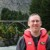# 2^2 Factorial Design with Center Points – Curvature Detected

Six Sigma – iSixSigma Forums General Forums Methodology 2^2 Factorial Design with Center Points – Curvature Detected

Viewing 5 posts - 1 through 5 (of 5 total)
• Author
Posts
• #241693ce_seek
Participant

I am using a factorial design (2 factors 2 levels) with center points. After running ANOVA, I found the following:

1. Main factors were not significant. Only the interaction and the curvature were significant. What should be the model for this? Should I keep all the terms or should I only be keeping the interaction term? y = -0.144 + 0.3177A + 0.1662B – 0.04065AB
2. If curvature is detected, how will I proceed? Do I still need to check where the curvature lies? or would this be part of my recommendation for future studies?

Thank you!

0
#241697Robert Butler
Participant

There are two schools of thought concerning main effects and interactions – one school says if the interaction is significant you need to keep the main effects and the other one says you don’t.

As for the curvature:

a) I don’t see that term in your model

b) Assuming the equation is a typo – the curvature is generic which means all you know is that it exists therefore you will need to augment your design with one or perhaps two more experimental runs to identify the variable associated with the curvilinear behavior.

Something worth considering:

Take your two variables and scale them from -1 to 1 and re-run the analysis.

In order to do this you will need to find the actual minimum and maximum settings for each of the two variables and then do the following for each of the two variables.

A = (maximum + minimum)/2

B = (maximum – minimum)/2

Scaled X1 = (X1 – A1)/B1

Scaled X2 = (X2 – A2)/B2

If you re-run the analysis using the scaled terms you will get an equation that will allow you to directly compare the effects of a unit change in X1, X2, the generic squared term, and X1*X2 interaction.  What this buys you is the ability to choose between the two methods of final terms in the model.

For example, if the coefficients of the scaled X1 and X2 are small relative to the coefficient of X1*X2 then, since X1 and X2 alone are not significant nothing much will be lost in the way of model use.  On the other hand if they are equivalent then you might want to consider keeping them in the model.  Of course, all of this assumes you are also doing the usual things one should be doing with respect to assessing the differences in the two models – residual analysis, prediction accuracy, etc.

0
#241827ce_seek
Participant

Hi Robert,

I was actually waiting for your response. Thank you very much! Yes, I actually removed the ctr point term in my equation. This is the resulting regression equation. However, I am not sure how the term for the ct. point would be of use.

Y= -0.144 + 0.3177 A + 0.1662 B – 0.04065 AB + 0.560 Ct Pt

I have also read in other post that when there is a curvature, you just remove the term for curvature in the equation. It just indicates that there is a curvilinear behavior.

Thank you.

0
#241834Robert Butler
Participant

Well, there’s more to it than that.  It is correct that you cannot use the generic curvilinear expression for purposes of prediction because there isn’t any way to assign that response to either of the two variables you have.  This means, for purposes of prediction, the best you can do is an equation with mains and interaction.  However, you don’t just remove the term and forget about what it is telling you.

If you are planning on using the equation you can construct for prediction purposes then there are several things you need to do in order to give yourself some understanding of the risks you are taking by using a predictive equation that has not accounted for all of the special cause variability in your data.

1. Residual plots – look at the residuals against the predicted – how far away from the zero line are the data points associated with the center points?  If the distance is “large” (yes, this is a judgement call) then you could go wrong with great assurance.  On the other hand if the points associated with the center points are “close to” but still on one side or the other then you are still not accounting for all of the special cause but, if you can’t do any more with respect to a few augmentation runs, it would be worth testing the equation to see how well it performs.

2. You need to scale and center your X’s in the manner I outlined in my first post and rerun the regression.  As I said, when you do this you will have a way to directly compare the effects of unit changes in the terms in the model.

Just looking at your equation based on the raw numbers my guess is that the units of X1 and X2 are large which would account for the small coefficient for the interaction.  I’m not sure what to make of the large coefficient for the generic squared term.  If you re-run the analysis with the scaled X1 and X2 you will be able to directly assess the impact of choosing to ignore the existence of curvature.

0
#241937Chris Seider
Participant

Did you have enough gap between your levels for A and B?  This (too small of change in X’s) can cause small enough difference in your Y such that you can’t get statistical confidence.

0
Viewing 5 posts - 1 through 5 (of 5 total)

You must be logged in to reply to this topic.